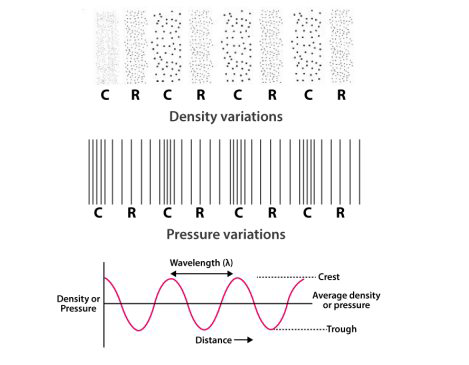# NCERT Exemplar Solutions Class 9 Science Solutions for Sound - Exercise in Chapter 12 - Sound

Question 20 Sound - Exercise

Draw a curve showing density or pressure variations with respect to distance for a disturbance produced by sound. Mark the position of compression and rarefaction on this curve. Also define wavelengths and time period using this curve.Video transcript
"hello everyone welcome to leader learning i am gurpreet your science tutor today's question is draw a curve showing density of pressure variations with respect to distance of a disturbance produced by the sun mark the position and compression of rear of compression and rear fraction on this curve also define wavelengths and the time period using the curve so here a figure is shown you can see this shows that density variation the first one then is your pressure variation and the curve which shows density of pressure variations with respect to the distance of a disturbance so here is a curve the distance between the two crest is known as the wavelength here right so this is the required figure for the answer for more such videos please subscribe leader learning and for any doubts drop a comment thank you"
Related Questions
Exercises

Lido

Courses

Teachers

Book a Demo with us

Syllabus

Maths
CBSE
Maths
ICSE
Science
CBSE

Science
ICSE
English
CBSE
English
ICSE
Coding

Terms & Policies

Selina Question Bank

Maths
Physics
Biology

Allied Question Bank

Chemistry
Connect with us on social media!